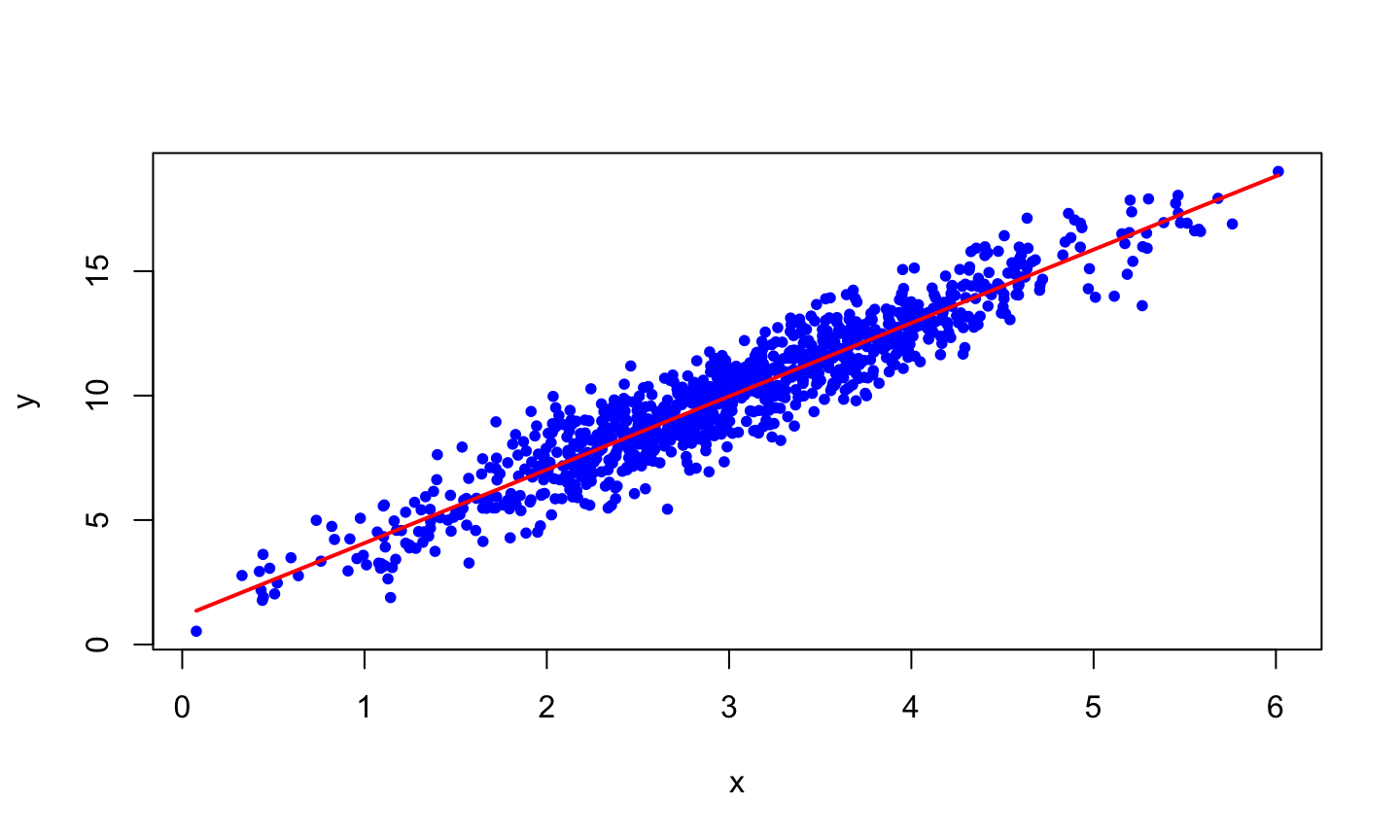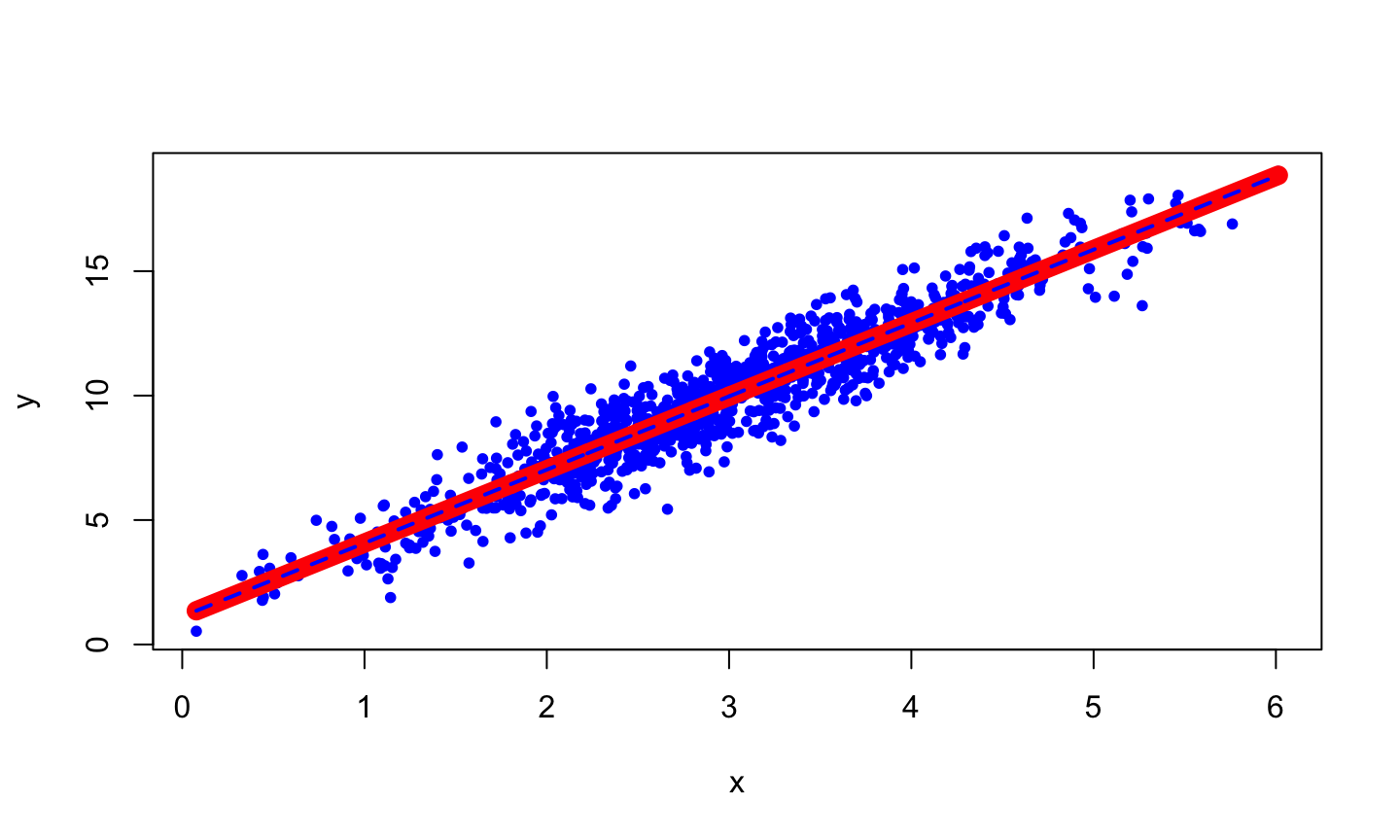School of Economics and Management
Beihang University
http://yanfei.site

Many problems in business require something to be minimized or maximized.

• Maximizing Revenue
• Minimizing Costs
• Minimizing Delivery Time
• Maximizing Financial Returns

Optimisation in Statistics

• Maximum Likelihood
• Least Squares
• Method of Moments
• Posterior Mode

Optimisation

• Suppose we want to find a minimum or maximum of a function $$f(x)$$.
• Sometimes $$f(x)$$ will be very complicated.
• Are there computer algorithms that can help?
• Yes!

Root Finding

• Consider the problem of finding the root of a function.
• For the function $$f(x)$$, the root is the point $$x^*$$ such that $$f(x^*) = 0$$.
• An algorithm for solving this problem was proposed by Newton and Raphson nearly 500 years ago.

Newton-Raphson Method

• Newton-Raphson is an iterative method that begins with an initial guess of the root.
• The method uses the derivative of the function $$f^{'}(x)$$ as well as the original function $$f(x)$$.
• When successful, it converges (usually) rapidly, but may fail as any other root-finding algorithm.

Newton-Raphson Method

The method tries to solve an equation in the form of $$f(x) = 0$$ through the iterative procedure: $x_{n+1} = x_n - \frac{f(x_n)}{f^{'}(x_n)}.$

Geometric interpretationStopping Rule

• With each step the algorithm should get closer to the root.
• However, it can run for a long time without reaching the exact root.
• There must be a stopping rule otherwise the program could run forever.
• Let $$\epsilon$$ be an extremely small number e.g. $$1 \times 10^{-10}$$ called the tolerance level.
• If $$|f(x^{*})| < \epsilon$$ then the solution is close enough and there is a root at $$x^{*}$$.

Example

• Now find the root of $$f(x) = x^2 - 5$$ using Newton method by hand.
• Tell about its geometric interpretation.

Newton-Raphson Method

1. Select an initial guess $$x_0$$, and set $$n = 0$$.
2. Set $$x_{n+1} = x_n - \frac{f(x_n)}{f^{'}(x_n)}$$.
3. Evaluate $$|f(x_{n+1})|$$.

1. If $$|f(x_{n+1})| < \epsilon$$, then stop;
2. Otherwise set $$n=n+1$$ and go back to step 2.

Lab Session 5

1. Write R code to find the root of $$x^2 = 5$$.
2. Now use your Newton-Raphson code to find the root of $$f(x) = \sqrt{|x|}$$. Try initial value 0.25.
3. Now use your Newton-Raphson code to find the root of $$x e^{-x^2} = 0.4(e^x + 1)^{-1} + 0.2$$. Try initial values 0.5 and 0.6.

What did you learn from mistakes?

You can use D()

newton.raphson <- function(f, init.value, df = NULL, tol = 1e-5, maxiter = 1000) {
if (is.null(df)) {
df <- D(f, 'x')
}
niter <- 0
diff <- tol + 1
x <- init.value
while (diff >= tol && niter <= maxiter) {
niter <- niter + 1
fx <- eval(f)
dfx <- eval(df)
if (dfx == 0) {
warning("Slope is zero: no further improvement possible.")
break
}
diff <- -fx/dfx
x <- x + diff
diff <- abs(diff)
}
if (niter > maxiter) {
warning("Maximum number of iterations 'maxiter' was reached.")
}
return(list(root = x, f.root = fx, niter = niter, estim.prec = diff))
}

You can also use deriv()

newton.raphson <- function(ftn, x0, tol = 1e-9, max.iter = 100) {
# Newton_Raphson algorithm for solving ftn(x) == 0
# we assume that ftn is a function of a single variable that returns
# the function value and the first derivative as a vector of length 2
#
# x0 is the initial guess at the root
# the algorithm terminates when the function value is within distance
# tol of 0, or the number of iterations exceeds max.iter

# initialise
x <- x0
fx <- ftn(x)
iter <-  0

# continue iterating until stopping conditions are met
while ((abs(fx) > tol) && (iter < max.iter)) {
x <- x - fx/fx
fx <- ftn(x)
iter <-  iter + 1
cat("At iteration", iter, "value of x is:", x, "\n")
}

# output depends on success of algorithm
if (abs(fx) > tol) {
cat("Algorithm failed to converge\n")
return(NULL)
} else {
cat("Algorithm converged\n")
return(x)
}
}

f <- expression(x^2 - 5)
df <- deriv(f, 'x', func = TRUE)
ftn <- function(x){
dfx <- df(x)
f <- dfx
}

newton.raphson(ftn, 1)

Other Examples

Now try these functions:

1. $$f(x) = x^3 - 2x^2 - 11x + 12$$, try starting values 2.35287527 and 2.35284172.
2. $$f(x) = 2x^3 + 3x^2 + 5$$, try starting values 0.5 and 0.

Learn from Mistakes

• Newton-Raphson does not always converge.
• For some functions, using some certain starting values leads to a series that converges, while other starting values lead to a series that diverges.
• For other functions different starting values converge to different roots.
• Be careful when choosing the initial value.
• Newton-Raphson doesn’t work if the first derivative is zero.

Conclusion

• Why did we spend so much time on finding roots of an equation?
• Isn’t this topic meant to be about optimization?
• Can we change the algorithm slightly so that it works for optimization?

Finding a Maximum or Minimum

• Suppose we want to find an minimum or maximum of a function $$f(x)$$ (think about maximum likelihood estimation).
• Find the derivative $$f^{'}(x)$$ and find $$x^{*}$$ such that $$f^{'}(x^*) = 0$$.
• This is the same as finding a root of the first derivative. We can use the Newton Raphson algorithm on the first derivative.

Newton Raphson algorithm for finding local maxima or minima

1. Select an initial guess $$x_0$$, and set $$n = 0$$.
2. Set $$x_{n+1} = x_n - \frac{f^{'}(x_n)}{f^{''}(x_n)}$$.
3. Evaluate $$|f^{'}(x_{n+1})|$$.

1. If $$|f^{'}(x_{n+1})| < \epsilon$$, then stop;
2. Otherwise set $$n=n+1$$ and go back to step 2.

Different Stopping Rules

Three stopping rules can be used.

• $$|f^{'}(x_{n+1})| < \epsilon$$.
• $$|x_{n} - x_{n-1}| < \epsilon$$.
• $$|f(x_{n}) - f(x_{n-1})| < \epsilon$$.

Intuition

• Focus the step size $$-\frac{f'(x)}{f''(x)}$$.
• The signs of the derivatives control the direction of the next step.
• The size of the derivatives control the size of the next step.
• Consider the concave function $$f(x)=-x^4$$ which has $$f'(x)=-4x^3$$ and $$f''(x)=-12x^2$$. There is a maximum at $$x^{*}=0$$.

Role of first derivativeRole of first derivativeRole of first derivativeRole of first derivative

• If $$f''(x)$$ is negative the function is locally concave, and the search is for a local maximum.
• To the left of this maximum $$f'(x)>0$$.
• Therefore $$-\frac{f'(x)}{f''(x)}>0$$.
• The next step is to the right.
• The reverse holds if $$f'(x)<0$$.
• Large absolute values of $$f'(x)$$ imply a steep slope. A big step is needed to get close to the optimum. The reverse hold for small absolute value of $$f'(x)$$.

Role of first derivative

• If $$f''(x)$$ is positive the function is locally convex, and the search is for a local minimum.
• To the left of this maximum $$f'(x)<0$$.
• Therefore $$-\frac{f'(x)}{f''(x)}>0$$.
• The next step is to the right.
• The reverse holds if $$f'(x)>0$$.
• Large absolute values of $$f'(x)$$ imply a steep slope. A big step is needed to get close to the optimum. The reverse hold for small absolute value of $$f'(x)$$.

Role of second derivativeRole of second derivative

• Together with the sign of the first derivative, the sign of the second derivative controls the direction of the next step.
• A larger second derivative (in absolute value) implies a larger curvature.
• In this case smaller steps are need to stop the algorithm from overshooting.
• The opposite holds for a small second derivative.

Functions with more than one input

• Most interesting optimization problems involve multiple inputs.
• In determining the most risk efficient portfolio the return is a function of many weights (one for each asset).
• In least squares estimation for a linear regression model, the sum of squares is a function of many coefficients (one for each regressor).
• How do we optimize for functions $$f({x})$$ where $${x}$$ is a vector?

Derviatives

• Newton's algorithm has a simple update rule based on first and second derivatives.
• What do these derivatives look like when the function is $$y=f({x})$$ where $$y$$ is a scalar and $$\mathbf{x}$$ is a $$d\times 1$$ vector?

First derivative

Simply take the partial derivatives and put them in a vector $\frac{\partial y}{\partial{\mathbf{x}}}= \left( \begin{array}{c} \frac{\partial y}{\partial x_1}\\ \frac{\partial y}{\partial x_2}\\ \vdots\\ \frac{\partial y}{\partial x_d} \end{array} \right)$ This is called the gradient vector.

An example

The function $$y=x_1^2-x_1x_2+x_2^2+e^{x_2}$$ has gradient vector

$\frac{\partial y}{\partial{\mathbf{x}}}=\left(\begin{array}{c} 2x_1-x_2\\ -x_1+2x_2+e^{x_2} \end{array} \right).$

Second derivative

Simply take the second order partial derivatives. This will give a matrix $\frac{\partial y}{\partial{\mathbf{x}}\partial{\mathbf{x}}'}= \left( \begin{array}{cccc} \frac{\partial^2 y}{\partial x_1^2}&\frac{\partial^2 y}{\partial x_1\partial x_2}&\cdots&\frac{\partial^2 y}{\partial x_1\partial x_d}\\ \frac{\partial^2 y}{\partial x_2\partial x_1}&\frac{\partial^2 y}{\partial x_2^2}&\cdots&\frac{\partial^2 y}{\partial x_2\partial x_d}\\ \vdots&\vdots&\ddots&\vdots\\ \frac{\partial^2 y}{\partial x_d\partial x_1}&\frac{\partial^2 y}{\partial x_d\partial x_2}&\cdots&\frac{\partial^2 y}{\partial x_d^2}\\ \end{array} \right).$

This is called the Hessian matrix.

An example

The function $$y=x_1^2-x_1x_2+x_2^2+e^{x_2}$$ has Hessian matrix $\frac{\partial y}{\partial{\mathbf{x}}\partial{ \mathbf{x}}'}=\left(\begin{array}{cc} 2 & -1\\ -1 & 2 + e^{x_2} \end{array} \right)$

Newton’s algorithm for multidimensional optimization

We can now generalise the update step in Newton's method: $\mathbf{x_{n+1}}=\mathbf{x_n}-\left(\frac{\partial^2 f({ \mathbf{x}})}{\partial {\mathbf{x}}\partial{\mathbf{x}}'}\right)^{-1} \frac{\partial f({\mathbf{x}})}{\partial {\mathbf{x}}}$

Now write code to minimise $$y=x_1^2-x_1x_2+x_2^2+e^{x_2}$$.

Maximum likelihood Estimate for linear models

Assume you want to make a regression model $y_i = \beta_0 + \beta_1x_{i} + \epsilon_i,~\text{where}~\epsilon_i \sim N(0, 1).$

• How do we estimate the parameters?
• Write down the log likelihood function with respect to the unknown parameters.
• Write down the gradient for the log likelihood function.
• Write down the Hessian for the log likelihood function.
• Use your newton function to obtain the best parameter estimate.

Optimizing Using Newton's Method

## Generate some data
beta0 <- 1
beta1 <- 3
sigma <- 1
n <- 1000
x <- rnorm(n, 3, 1)
y <- beta0 + x * beta1 + rnorm(n, mean = 0, sd = sigma)
# plot(x, y, col = "blue", pch = 20)

## Make the log normal likelihood function
func = function(beta){
sum((y-beta-beta*x)^2)
}

matrix(c(sum(-2*(y-beta-beta*x)),sum(-2*x*(y-beta-beta*x))),2,1)
}

hess = function(beta){
matrix(c(2*length(x),2*sum(x),2*sum(x),2*sum(x^2)),2,2)
}

## The optimization
source('./RCode/newton.R')
optimOut <- newton(function(beta){list(func(beta), grad(beta), hess(beta))}, c(1.1, 1.3))
beta0Hat <- optimOut
beta1Hat <- optimOut
yHat <- beta0Hat + beta1Hat * x

## Plot
plot(x, y, pch = 20, col = "blue")
points(sort(x), yHat[order(x)], type = "l", col = "red", lwd = 2)Comparison with OLS

myLM <- lm(y~x)
myLMCoef <- myLM\$coefficients
yHatOLS <- myLMCoef + myLMCoef * x
plot(x, y, pch = 20, col = "blue")
points(sort(x), yHat[order(x)], type = "l", col = "red", lwd = 10)
points(sort(x), yHatOLS[order(x)], type = "l",
col = "blue", lty="dashed", lwd = 2, pch = 20)Lab Session 7

Use Newton’s method to find the maximum likelihood estimate for the coefficients in a logistic regression. The steps are:

1. Write down likelihood function.
2. Find the gradient and Hessian matrix.
3. Code these up in R.
4. Simulate some data from a logistic regression model and test your code.